# Excel tip: Find missing fractional cents

## Microsoft Excel consultant Bill Jelen, aka MrExcel, demonstrates how an auditor can locate fractional cents hidden by the Decrease Decimal icon.

Microsoft Excel offers calculation precision to 15 digits. While this might be useful for scientific applications,...

if you are simply trying to create a sales invoice, you really only need two decimal places after the decimal. When your sales invoice shows fractional cents, many people will use the Decrease Decimal icon to ensure that the numbers only show two decimal places.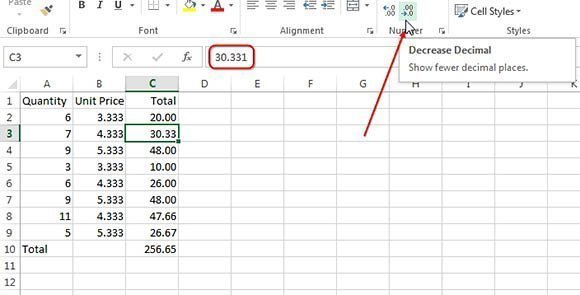While this makes the invoice look cleaner, it introduces a new problem. The numeric formatting in Excel does not change how the underlying numbers are stored. It simply changes how the numbers are displayed.

If someone would use a calculator or even spot-checks the numbers, the total will be wrong. In this figure, it would be easy for any eagle-eyed accounts payable worker to scan the final digits of column C, add the 3+6+7 and conclude that the invoice total should end in 6 instead of the 5 shown on the invoice.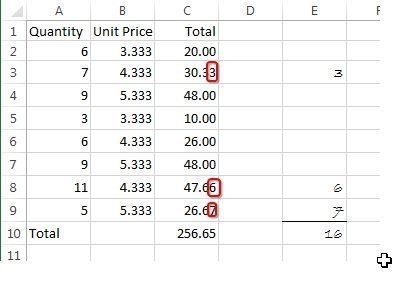If you are an auditor and need to find the missing fractional cents, you could build a formula where you subtract C2-ROUND(C2,2). Be sure to use the Increase Decimal icon so you can see the places where the fractional pennies are off.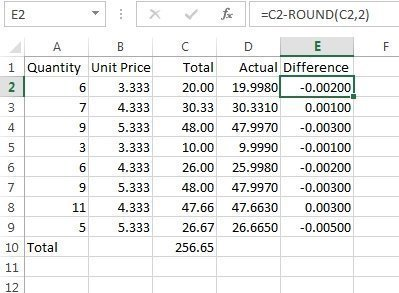One quick but dangerous solution is to use the "Set precision as displayed" checkbox. To find this checkbox, go to File, Options, Advanced, then scroll 90% of the way through the long list of options. In the section for "When calculating this workbook," it is the second checkbox. This setting will change how Excel internally stores the numbers to match how they are currently displayed.

This would be a great setting if you could apply it only to a selected range. But the setting applies to all cells in all worksheets of the workbooks, and therefore might cause other calculation errors.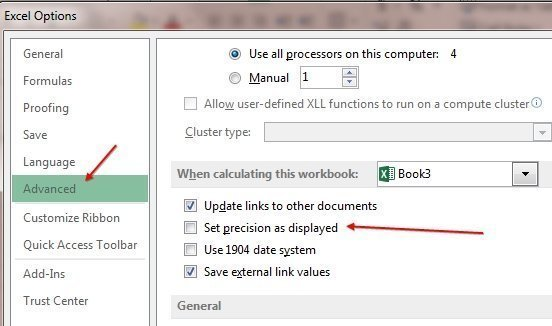A better solution is the ROUND() function. Had the person designing the worksheet used =ROUND(B2*C2,2) in column D instead of =B2*C2, the problem would never have happened in the first place.

One unusual way to solve the problem after the fact is to ask Excel to sum the rounded version of each number in the range. Instead of using =ROUND(SUM(C2:C9,2), you can use a formula of =SUM(ROUND(C2:C9,2)). This is a special type of formula called an array formula. After typing the formula, rather than pressing Enter, you must hold down Ctrl+Shift while pressing Enter. You will know you did this correctly if you see curly braces appear around the formula in the formula bar.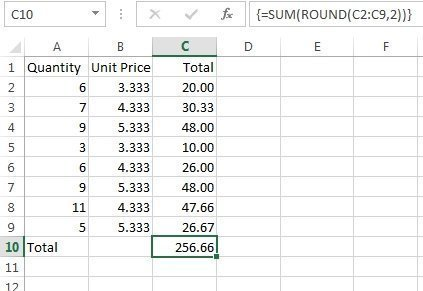It is important to note that you do not type the curly braces. They are displayed by Excel to indicate that it is an array formula. Any time you edit the formula, you must confirm the edits with Ctrl+Shift+Enter, or the formula will revert to a #VALUE! error.